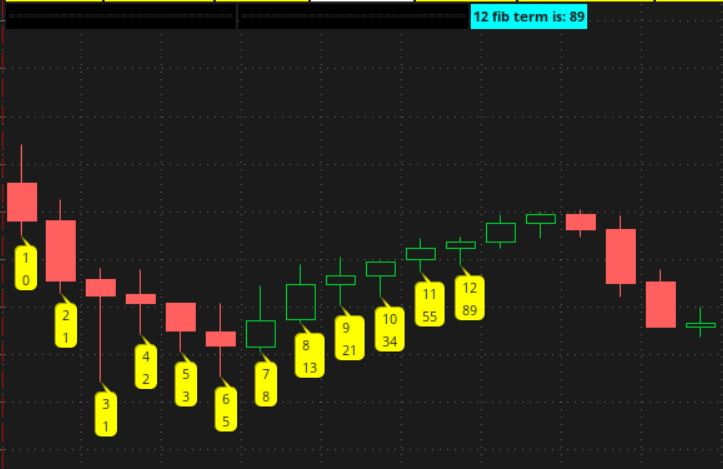# Find the Fibonacci Sequence nth term In ThinkOrSwim

#### halcyonguy

##### Well-known member
VIP
Find the Fibonacci Sequence nth term In ThinkOrSwim
i made this with the intention that the sub script could be used in other projects. by itself, it is of no use for trading.
(someone asked for an average, weighted with fib series numbers, and this could be used for that project)

this study will return that nth term, in the Fibonacci sequence.
0, 1, 1, 2, 3, 5, 8, 13, 21, 34,...

ex. the 5th term is 3

it will,
. display the nth term number in a label
. draw bubbles with, the barnumber and the series number, up to the desired nth number

Ruby:
``````# fibonacci_series_nth_term_01

#  calc the nth term in a fibonacci series

# https://math.hmc.edu/funfacts/fibonacci-number-formula/
#  the sequence begins: 0, 1, 1, 2, 3, 5, 8, 13, 21, 34, ...
#  the formula for the n-th term is:
#  p1 = (1 + Sqrt) / 2
#  p2 = (1 – Sqrt) / 2
#  nterm = ( p1^n – p2^n ) / Sqrt(5)
#--------------------------

script fibnum {
input term = 1;
# adjust the input number, so the first output is 0
def n = term - 1;

def p1 = (1 + Sqrt(5))/2;
def p2 = (1 – Sqrt(5))/2;
def fterm = ( power(p1 , n) – power(p2, n ) ) / Sqrt(5);

plot fibvalue = fterm;
}

# ---------------------------
#  main
# ---------------------------
def na = double.nan;
def bn = BarNumber();
input show_fib_terms = yes;
#--------------------
# test1 , show nth fib number in a label
input fib_term = 12;
def f1 = fibnum(fib_term);
AddLabel( show_fib_terms, fib_term + " fib term is: " + f1, Color.CYAN);
#--------------------
# test2 , show fib #'s in bubbles on the first few candles
def calcfib = (bn <= fib_term);
def fib_index = if calcfib then bn else na;
def f2 = if !calcfib then na else fibnum(fib_index);
AddChartBubble(  show_fib_terms and calcfib, low, bn + "\n" + f2, Color.YELLOW, no);
#-------------------
#``````Last edited:
Hi,

I have been looking for a script similar to your concept but instead of labeling each candle, it will only label the candle according to the sequence. for example Candle # 4,6,7 will be skipped but candles 1,2,3,5,8,13,21 will be labeled or colored. Also it be can anchored to a certain starting period and have the fib sequence candle with a unique color. Please let me know if this is possible.

### Not the exact question you're looking for?

87k+ Posts
389 Online## The Market Trading Game Changer

Join 2,500+ subscribers inside the useThinkScript VIP Membership Club
• Exclusive indicators
• Proven strategies & setups
• Private Discord community
• Exclusive members-only content
• 1 full year of unlimited support

What is useThinkScript?

useThinkScript is the #1 community of stock market investors using indicators and other tools to power their trading strategies. Traders of all skill levels use our forums to learn about scripting and indicators, help each other, and discover new ways to gain an edge in the markets.

How do I get started?

We get it. Our forum can be intimidating, if not overwhelming. With thousands of topics, tens of thousands of posts, our community has created an incredibly deep knowledge base for stock traders. No one can ever exhaust every resource provided on our site.

If you are new, or just looking for guidance, here are some helpful links to get you started.

What are the benefits of VIP Membership?